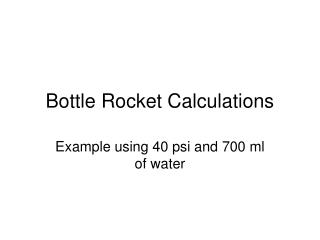DownloadDownload PresentationBottle Rocket Calculations

# Bottle Rocket Calculations

Download Presentation## Bottle Rocket Calculations

- - - - - - - - - - - - - - - - - - - - - - - - - - - E N D - - - - - - - - - - - - - - - - - - - - - - - - - - -
##### Presentation Transcript

1. Bottle Rocket Calculations Example using 40 psi and 700 ml of water

2. Select air pressure and water volume • Select air pressure i.e. 40 psi • Select water volume i.e. 700 ml • Find mass of water • Mass = density x volume • Vol = 700 ml = 700 cm3 or 0.000700 m3 • ρ = density of water = 998 kg/m3 which is a constant • Mass = 998 kg/m3 x 0.0007 m3 = 0.6986 kg

3. Calculate average water mass flow rate • Average mass flow rate, ṁ, of water out of nozzle: ṁ = A X cd X √(2ρΔP) • Find A of nozzle in m2: A = πr2 • For diameter of ~21 cm = .021 m • Radius = d/2 = .021 m / 2 = 0.0105 m • A = π (0.0105)2 = 0.0003462 m2 • Cd is given at 0.98

4. (water mass flow rate cont.) • Find average pressure acting on the water • ΔP = (Pi + Pf) / 2 or (Pi (1+Vi/Vf)) / 2, since PiVi = PfVf, so Pf = (PiVi)/Vf • Pi = 40 psi • Vi of air = 2 Liter – 0.7 L = 1.3 L • Vf = 2 L • Pf = 40 (1.3) / 2 = 26 • ΔP = (40 + 26) / 2 = 33psi • Convert psi to N/m2 • 14.7 psi = 101,353.56 N/m2, so 33 psi = • 101,353.56 x 33 / 14.7 = 227528.4 N/m2 • Note: N = kg m/s2

5. (water mass flow rate cont.) • Back to calculating average mass flow rate, ṁ, of water out of nozzle: ṁ = A x cd x √(2ρΔP) • ṁ = A x cd x √(2ρΔP) • ṁ = 0.0003462 m2 x 0.98 x (√2 x 998 kg/m3 x 227528.4 N/m2) = 7.2302 kg/s

6. Water Exit Velocity & Thrust • Water exit velocity V = ṁ / ρA = 7.2302 kg/s / (998 kg/m3)(.0003462 m2) = 20.926 m/s • Rocket thrust ft = ṁ x V = 7.2302 kg/s x 20.926 m/s = 151.3 kg m/s2 or 151.3 N

7. Net Force on Rocket • Net force f = ft – fd – (mave x g) • F = 151.3 kg m/s2 – 0 – ((0.3 + 0.7 kg)/2) x (9.8 m/s2) = 146.4 kg m/s2 or 146.4 N • Note: • Mave = mass of empty rocket + mass of water selected • Mass of rocket was weighted at 300 g, or 0.3 kg • Water selected was 0.7 kg • fd is the drag coefficient and is very low in this case

8. Rocket Acceleration • The rocket acceleration is a result of the net force acting on the mass • f = mave x a, so a = f/ mave • A = (146.4 N) / ((0.3 + 0.7 kg)/2) = 292.8 m/s2

9. Range • To find the range you need to find the amount of time it takes for the water to exit and the velocity of the rocket • The time to expel the water is the mass of the water divide by the mass flow rate: t = m H2O/ ṁ = 0.7 kg / 7.2302 kg/s = 0.097 s

10. (Range cont.) • Velocity of the bottle Vrocket is a x t = • (292.8 m/s2) x (0.097 s) = 28.4 m/s • So the range R is V2rocket x sin 2Ө / g • Ө is the launch angle which is 45° in this case. • R = (28.4 m/s)2 x sin 2(45) / 9.8 m/s2 = 82.3 m

11. Final Range • Final range is affected by drag factor • Drag factor, Dc, for bottle shape is low, i.e. 0.15 • Drag force D = 1- Dc = 1 – 0.15 = 0.85 • Final range Rf = R x D = 82.3 m x 0.85 = 69.96 m